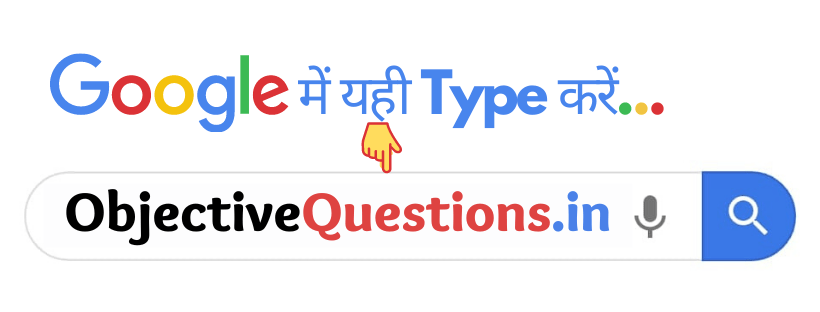# Functions

1. A function cannot be defined inside –

• Another function
• A program
• A class
• None of these
Another function

2. Which C libram file declare functions for processing strings ?

•  < Stdlib.h >
•  < String.h >
•  < time.h >
•  < Stdio.h >
< String.h >

3. Not initializing a reference variable will cause :

• Termination of program
• A Syntax error
•  Nothing
A Syntax error

4. Int & x ; this syntax is used when :

•  X is passed by reference
• X is declared outside the function
• X is passed by value
•  None of the above
X is passed by reference

5. Standard C library file < stdib.h ) is used for :

• Declares data function
• Declares time function
• Declares a mathematical function
• Declares a utility function
Declares a utility function

6. Iteration used a repetition structure whereas recursion uses :

• Selection Structure
• Controlling Structure
•  Sorting Structure
• All of these
Selection Structure

7. A function is invoked by a :

• Operator call
• An object call
• Function call
• None of these
Function call

8. Which C ++ function is used for finding absolute values?

• Expo
• Fabs
•  atan
•  Pow
Fabs

9. Pre-defined function and other program elements are accessed through :

•  Classes
• Operator
• All of these

10. In which circumstances recursion function is called?

• For calling maths function
•  To calling input and output stream
• To solve a problem
• All of the above
To solve a problem

11. Void function is also called as :

• Subroutine
• Procedure
• Both a and b
• Terminator
Both a and b

12. Which function is known as the standard library function?

• Void
• Sort
•  Maths
• sqrt
Maths

13. Strand function is used for :

•  Including the timestamps
• Getting randomizing effect
• Calling a maths function
•  None of these
Getting randomizing effect

14. Like swap ( ) function if we want to change the value of the actual parameter we must call parameters :

• By reference
•  By value
• Outside the function
• None of these
By reference

15. INT – MAX Constant is defined in which header file?

•   < time .h
•  < math.h >
• < limits .h
•  Stdlib.h
< limits .h

16. Recursive alt is also known as

• Operator call
•  Recursion step
• Complex calls
•  None of these
Recursion step

17. Which of the following is not a maths function

• Pow ( )
• Log ( )
• Time ( )
• Expo ( )
Time ( )

18. Function body is also referred as :

•  Block
• Compound statement
• Class
•  All of these
Block

19. Default return type for every function is :

• String
• Float
• Char
• Int
Int

20. How many storage classes specifies are offered by C ++?

•  3
•  4
•  5
• 2
5

21. Which from following is not a storage class specifier in C++:

• Mat
• Extern
• Register
•  Auto
Mat

22. A function that needs no return value is called :

•  Finite function
• Void function
•  Infinite function
•  All of these